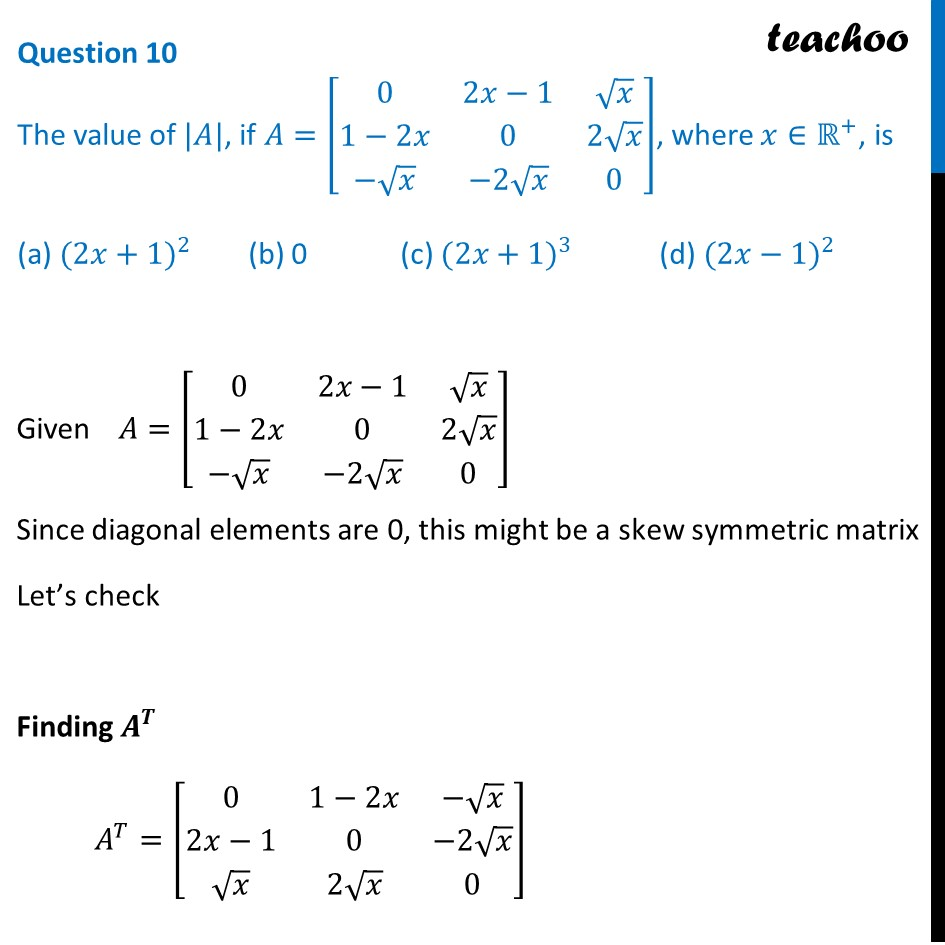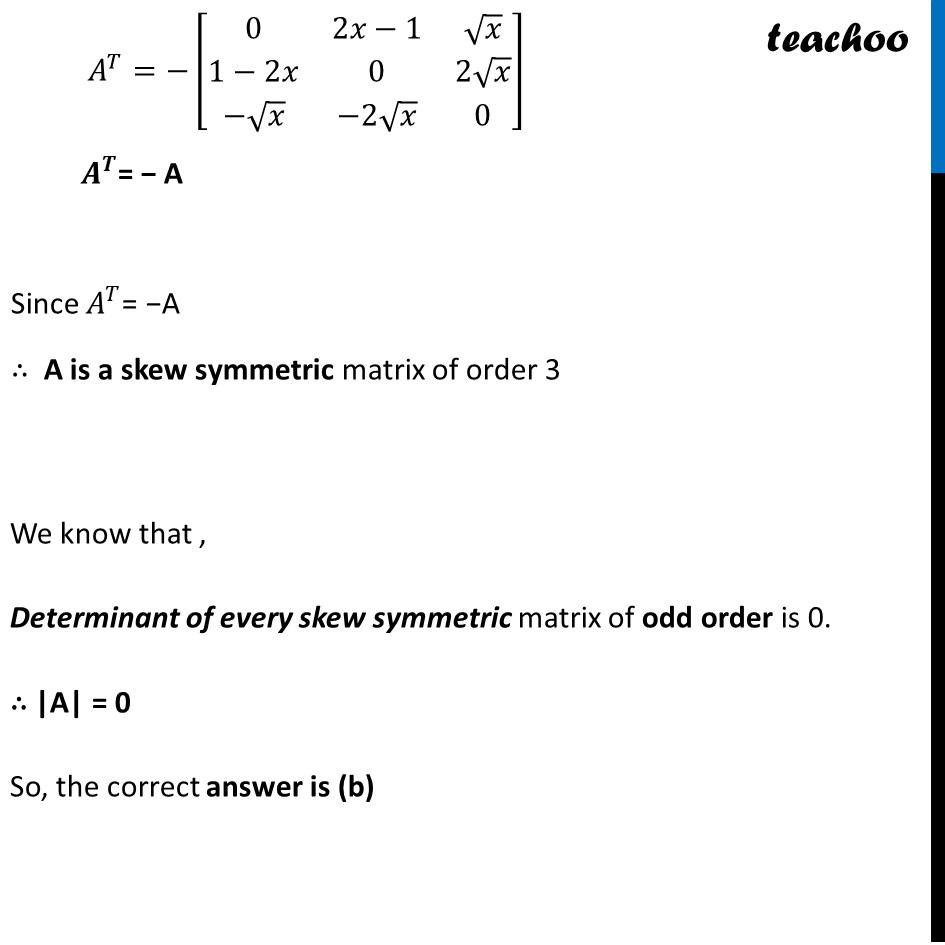CBSE Class 12 Sample Paper for 2024 Boards

Class 12
Solutions of Sample Papers and Past Year Papers - for Class 12 Boards

## (a) (2x+1)^2         (b) 0             (c) (2x+1)^3            (d) (2x-1)^2Learn in your speed, with individual attention - Teachoo Maths 1-on-1 Class

### Transcript

Given 𝐴=[■(0&2𝑥−1&√𝑥@1−2𝑥&0&2√𝑥@−√𝑥&−2√𝑥&0)] Since diagonal elements are 0, this might be a skew symmetric matrix Let’s check Finding 𝑨^𝑻 𝐴^𝑇=[■(0&1−2𝑥&−√𝑥@2𝑥−1&0&−2√𝑥@√𝑥&2√𝑥&0)] 𝐴^𝑇=−[■(0&2𝑥−1&√𝑥@1−2𝑥&0&2√𝑥@−√𝑥&−2√𝑥&0)] 𝑨^𝑻= − A Since 𝐴^𝑇= −A ∴ A is a skew symmetric matrix of order 3 We know that , Determinant of every skew symmetric matrix of odd order is 0. ∴ |A| = 0 So, the correct answer is (b)